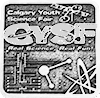SILVER#### Opposites Attract

This study examines what happens during an interaction between an apple made of antimatter and a desk made of matter.

### Problem

Antimatter is the opposite of normal matter (CERN, 2021a). The protons and electrons have their charges reversed, leading to positrons and antiprotons (CERN, 2021a). When an antiparticle comes into contact with a normal particle, they will annihilate, eradicating each other in a burst of energy (Sutton, 2016).

Theoretically, what would happen if an apple made of antimatter was placed on a table made of matter?

### Method

1. Background Research
• Performed background research about antimatter and collisions involving antimatter and matter.
• Identified the physical equation required to answer the question (Einstein’s Theory of Relativity, E = mc2).
2. Measurements
• Obtained the weight of an apple using a food scale.
1. Calculations
• Used the weight of an apple to represent it’s mass and multiplied it by two to estimate the mass of the antimatter and equal amount of matter during the anhilitation.
• Multiplied the total mass by speed of light squared.
• Calculated the amount of energy released in the explosion.

### Research

What is antimatter?

• Everything around us is made up of matter.
• Matter is made up of tiny particles called electrons (negatively charged), protons (positively charged), and neutrons (no charge).
• Antimatter was discovered by British physicist Paul Dirac from the equation x2 = 4 that could have an answer of x=2 or x= -2 (CERN, 2021a). That meant that there were particles with the opposite charge of normal ones and for every electron there would be a positron, or an antielectron (CERN, 2021a), and for every proton there would be an antiproton

Where do you find antimatter?

• A big mystery in antimatter and matter is that when the big bang happened, there should have been equal amounts of antimatter and matter created, but there is much more matter in the universe than antimatter (Exploratorium, 2000).  Why?
• Antiprotons are created or found in the antiproton decelerator, when they come from a proton beam that hits a block of metal. When they go into the antiproton decelerator, a ring of magnets, they are cooled and ready to be sent into antimatter experiments
(CERN, 2021b).
• The ALPHA group successfully stored an antihydrogen atom for the first time. An antihydrogen atom is a positron rotating around an antiproton (Preuss, 2010).

How does antimatter behave?

• Electrons and positrons were observed in a bubble chamber that made positive charged particles curl right and negative ones curl left, so there would be two spirals curling opposite that would collide and annihilate (Particle Data Group, 2014).
• When antimatter and matter come together they annihilate each other: destroying each other in a blast of gamma energy (Sutton, 2016).
• If an antihydrogen and a hydrogen atom collided in the room
you were in, you likely would not notice it and would be fine,
except for a miniscule amount of radiation (Scudder, 2017).

Einstein’s Theory of Relativity

• E= mc2 is Einstein's Equation of Relativity (Perkowitz, 2018). Energy equals mass times the speed of light squared. This theory proves that mass and energy are the same thing and can be changed to each other.
• To calculate the amount of energy in antimatter, you multiply the mass of the antimatter by the speed of light squared.

### Data

Mass of apple and equivalent mass of desk combined = 0.34 kg

E = mc2

E = 0.34 kg x (8.99 x 1016 m2/s2)

E = 3.1 x 1016 kgm2/s2

E = 3.1 x 1016 J

The amount of energy released in a collision of an apple made of antimatter and
equivalent amount of matter from the table is 3.1 x 1016 J.

### Conclusion

Conclusion

• If an apple made of antimatter with a mass of 0.175 kg collided with an equivalent mass of matter, the particles would annihilate, releasing 3.1 x 1016 J of energy.
• The energy released from this explosion is equivalent to the energy released in an explosion from 7, 190, 000 tonnes of TNT (trinitrotoluene) (Kyle's Converter, 2009).

Study Limitations

• Calculations in this study were made with the assumption that mass equals weight. This calculation would change on different planets because weight is relative to gravity.

Possible Extensions Of My Project

• How could you store antimatter without heavy machinery, and to that extent, inject it into someone to destroy a cancer cell.
• Could life exist made solely out of antimatter in a ecosystem made out of antimatter.

### Acknowledgement

Thank you to my mom, Lisa Baker, who helped me type this with a broken arm and helped keep me on track.